## Saturday, 9 August 2014

### chapter 5 Some Standard Limits 1

(A)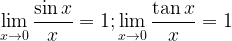$\mathop {\lim }\limits_{x \to 0} \dfrac{{\sin x}}{x} = 1;\mathop {\lim }\limits_{x \to 0} \dfrac{{\tan x}}{x} = 1$
Both the limits above are indeterminate, of the form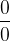$\dfrac{0}{0}$. We are discussing here a geometric interpretation of these limits. Consider a sector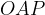$OAP$ of a unit circle as shown in the figure below.We see that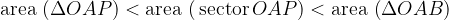${\rm{area}}\,\left( {\Delta OAP} \right) < {\rm{area}}\,\left( {{\,\rm{sector}}\,OAP} \right) < {\rm{area}}\,\left( {\Delta OAB} \right)$ or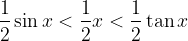$\dfrac{1}{2}\sin x< \dfrac{1}{2}x < \dfrac{1}{2}\tan x$ or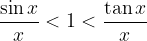$\dfrac{{\sin x}}{x} < 1 < \dfrac{{\tan x}}{x}$
What happens as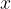$x$ decreases or as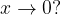$x \to 0?$
We see that the difference between the three areas considered above tends to decrease;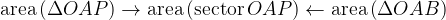${\rm{area}}\left( {\Delta OAP} \right) \to {\rm{area}}\left( {{\rm{sector}}\,OAP} \right) \leftarrow {\rm{area}}\left( {\Delta OAB} \right)$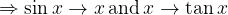$\Rightarrow \sin x \to x\,{\rm{and}}\,x \to \tan x$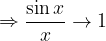$\Rightarrow \dfrac{{\sin x}}{x} \to 1$ (but remains less than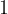$1$ )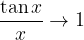$\dfrac{{\tan x}}{x} \to 1$ (remains greater than$1$ )$\Rightarrow \mathop {\lim }\limits_{x \to 0} \dfrac{{\sin x}}{x} = {1^ - };\mathop {\lim }\limits_{x \to 0} \dfrac{{\tan x}}{x} = {1^ + }$
Consider the limits below and you should understand:It is important to observe how any function approaches a limit. For example, in the case above, as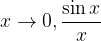$x \to 0,\dfrac{{\sin x}}{x}$ approaches$1$ from the left side while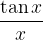$\dfrac{{\tan x}}{x}$approaches$1$ from the right side. This makes a big difference. Why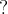$?$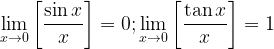$\mathop {\lim }\limits_{x \to 0} \left[ {\dfrac{{\sin x}}{x}} \right] = 0;\mathop {\lim }\limits_{x \to 0} \left[ {\dfrac{{\tan x}}{x}} \right] = 1$
(B)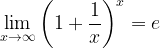$\mathop {\lim }\limits_{x \to \infty } {\left( {1 + \dfrac{1}{x}} \right)^x} = e$
Consider the expression for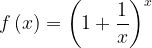$f\left( x \right) = {\left( {1 + \dfrac{1}{x}} \right)^x}$ . As$x$ gets larger and larger or as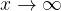$x \to \infty$ , the base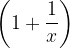$\left( {1 + \dfrac{1}{x}} \right)$ gets closer to$1$ while the exponent ($x$), tends to infinity. Hence, this limit is of the indeterminate form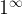${1^\infty }$. Its very important to get a ‘feel’ that the value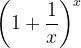${\left( {1 + \dfrac{1}{x}} \right)^x}$ will converge to a fixed, definite value, as$x$increases. You should get this feel by looking at the table below.$x$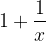$1 + \dfrac{1}{x}$${\left( {1 + \dfrac{1}{x}} \right)^x}$$1$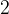$2$$2$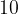$10$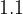$1.1$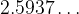$2.5937\ldots$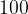$100$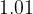$1.01$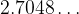$2.7048\ldots$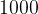$1000$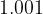$1.001$$2.7169\ldots$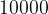$10000$$1.0001$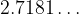$2.7181\ldots$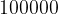$100000$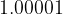$1.00001$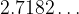$2.7182\ldots$
Try to show that the limit of${\left( {1 + \dfrac{1}{x}} \right)^x}$ is bounded and lies between and$2$ and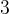$3$, that is,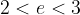$2 < e < 3$ (you can use the binomial theorem in conjunction with the sandwich theorem to prove this, by first proving it for an integral$x$)We see that as$x$ becomes larger, the term${\left( {1 + \dfrac{1}{x}} \right)^x}$ converges to some value (This can be proved) This limiting value is denoted by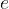$e$$e$ is an irrational number and its value is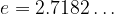$e = 2.7182\ldots$.
The limit we have just seen is extremely important and will be widely used subsequently.
Note another important point:
If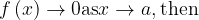$f\left( x \right) \to 0 {\rm{as}} x \to a,{\rm{then}}$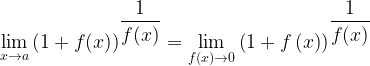$\mathop {\lim }\limits_{x \to a} {\left( {1 + f(x)} \right)^{\dfrac{1}{{f(x)}}}} = \mathop {\lim }\limits_{f(x) \to 0} {\left( {1 + f\left( x \right)} \right)^{\dfrac{1}{{f(x)}}}}$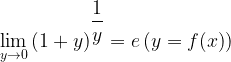$\mathop {\lim }\limits_{y \to 0} {\left( {1 + y} \right)^{\dfrac{1}{y}}} = e\left( {y = f(x)} \right)$
Hence, any limit of this form has the value$e$.